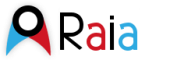## Dataset: LD

• Data format: netCDF
• Data type: GRID
• Naming Authority: cesam.ua.pt
• ID: romsLD

### Variables:

• Vocabulary [RHO]:
• h (meter) = bathymetry = m
• longitude = longitude = degree_east
• latitude = latitude = degree_north
• u (positive is eastward) = baroclinic eastward sea water velocity = m s-1
• v (positive is northward) = baroclinic northward sea water velocity = m s-1
• temp = temperature = Celsius
• salt (dimensionless) = salinity = PSU
• CHLA = Chlorophyll A concentration = mg Chla m-3
• Vocabulary []:
• time (gregorian) = time since initialization = seconds since 2013-1-2 00:00:00
• depth (meter) = Vertical distance below the surface = m

### GeospatialCoverage:

• Longitude: -12.5 to -5.5 degrees_east
• Latitude: 34.5 to 45.5 degrees_north
• Altitude: -1000.0 to 0.0 m (positive is up)